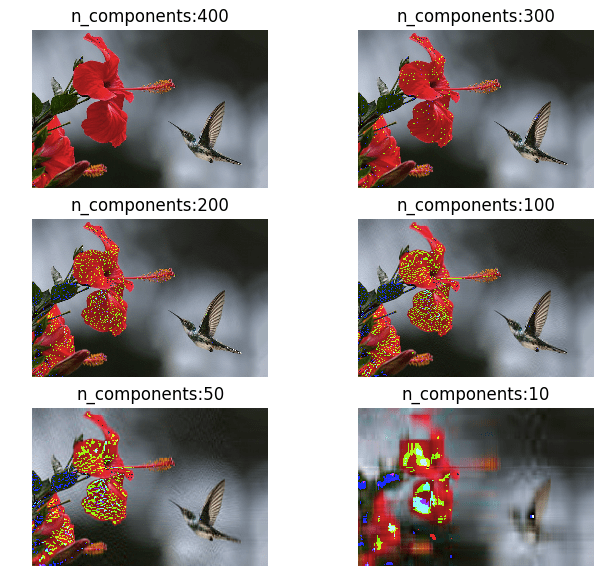# Data science

## K-fold cross validation

The greatest headache for any machine learning engineer is the problem of overfitting. The model we trained works perfectly on the training dataset but when applied to other new dataset it fails miserably. This is because of overfitting where our classifier learns the provided dataset accurately but fails when applied on new data. One good …

## Linear Regression using Gradient Descent Algorithm

Gradient descent is an optimization method used to find the minimum value of a function by iteratively updating the parameters of the function. Parameters refer to coefficients in Linear Regression and weights in Neural Networks. In a linear regression problem, we find a modal that gives an approximate representation of our dataset. In the below …## Mathematics of Principal component analysis

Principal component analysis is a method used to reduce the number of dimensions in a dataset without losing much information. It’s used in many fields such as face recognition and image compression, and is a common technique for finding patterns in data and also in the visualization of higher dimensional data. PCA is all about …

## Understanding the Classification report through sklearn

A Classification report is used to measure the quality of predictions from a classification algorithm. How many predictions are True and how many are False. More specifically, True Positives, False Positives, True negatives and False Negatives are used to predict the metrics of a classification report as shown below. The report is copied from our previous …

## K-Means on Iris Dataset

Read my previous post to understand how K-Means algorithm works. In this post I will try to run the K-Means on Iris dataset to classify our 3 classes of flowers, Iris setosa, Iris versicolor, Iris virginica (our classess) using the flowers sepal-length, sepal-width, petal-length and petal-width (our features) Getting data: import numpy as np import matplotlib.pyplot …

## Mathematics behind K-Mean Clustering algorithm

K-Means is one of the simplest unsupervised clustering algorithm which is used to cluster our data into K number of clusters. The algorithm iteratively assigns the data points to one of the K clusters based on how near the point is to the cluster centroid. The result of K-Means algorithm is: K number of cluster …

## Understanding Support vector Machines using Python

Support Vector machines (SVM) can be used for both classification as well as regression tasks but they are mostly used in classification applications. Some of the real world applications include Face detection, Handwriting detection, Document categorisation, SPAM Filtering, image classification and protein remote homology detection. For many researchers, SVM is the first best choice for …

## Simple example of Polynomial regression using Python

Previously I wrote an article explaining the underlying maths behind polynomial regression. In this post I will use Python libraries to regress a simple dataset to see polynomial regression in action. If you want to fully understand the internals I recommend you read my previous post. Polynomial regression is a method of finding an nth …

## Maths behind Polynomial regression

Polynomial regression is a process of finding a polynomial function that takes the form f( x ) = c0 + c1 x + c2 x2 ⋯ cn xn where n is the degree of the polynomial and c is a set of coefficients. Through polynomial regression we try to find an nth degree polynomial function which is the closest approximation of our data points. Below is a sample random dataset which has been regressed …

## Multiple Linear Regression with Python on Framingham Heart Study data

Previously we built a simple linear regression model using a single explanatory variable to predict the price of pizza from its diameter. But in the real world the price of pizza cannot be entirely derived from the diameter of its base alone. It also depends on the toppings, which means there are a many more …